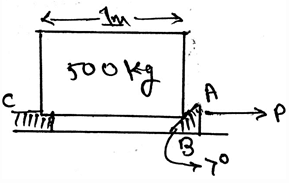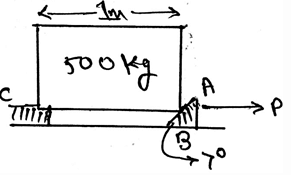Test: Wedges

# Test: Wedges

Test Description

## 15 Questions MCQ Test Engineering Mechanics | Test: Wedges

Test: Wedges for Mechanical Engineering 2022 is part of Engineering Mechanics preparation. The Test: Wedges questions and answers have been prepared according to the Mechanical Engineering exam syllabus.The Test: Wedges MCQs are made for Mechanical Engineering 2022 Exam. Find important definitions, questions, notes, meanings, examples, exercises, MCQs and online tests for Test: Wedges below.
Solutions of Test: Wedges questions in English are available as part of our Engineering Mechanics for Mechanical Engineering & Test: Wedges solutions in Hindi for Engineering Mechanics course. Download more important topics, notes, lectures and mock test series for Mechanical Engineering Exam by signing up for free. Attempt Test: Wedges | 15 questions in 30 minutes | Mock test for Mechanical Engineering preparation | Free important questions MCQ to study Engineering Mechanics for Mechanical Engineering Exam | Download free PDF with solutions
 1 Crore+ students have signed up on EduRev. Have you?
Test: Wedges - Question 1

### A ____________ is a simple machine that is used as to transfer applied forces into much larger forces.

Detailed Solution for Test: Wedges - Question 1

The main thing about the wedges is that the wedge is a simple machine that is used as to transfer applied forces into much larger forces. This means that the wedges are the means of force transfer. This machine is very useful in the industries, and it has a wide application.

Test: Wedges - Question 2

### Wedges are used to transfer heavy loads.

Detailed Solution for Test: Wedges - Question 2

The main thing about the wedges is that the wedge is a simple machine that is used as to transfer applied forces into much larger forces. This means that the wedges are the means of force transfer. This machine is very useful in the industries, and it has a wide application.

Test: Wedges - Question 3

### The blocks of heavy weights are being able to lift from the help of the wedges.

Detailed Solution for Test: Wedges - Question 3

The main thing about the wedges is that the wedge is a simple machine that is used as to transfer applied forces into much larger forces. This means that the wedges are the means of force transfer. This machine is very useful in the industries, and it has a wide application.

Test: Wedges - Question 4

The normal force exerted by the surface of the wedge is normal to the surface of the ________

Detailed Solution for Test: Wedges - Question 4

As we know the normal forces act perpendicular to the surfaces of the body kept over the wedges. Thus the normal force exerted by the surface of the wedge is normal to the surface of the base of the body kept over the wedge. The wedges are very useful in the industries, and it has a wide application.

Test: Wedges - Question 5

Which one is not the condition for the equilibrium in free body diagram for calculation of the normal forces along the body kept over the wedge, consider all forces to be straight and linear?

Detailed Solution for Test: Wedges - Question 5

For the equilibrium in the three dimensional system of axis we have all the conditions true as, ∑Fx=0, ∑Fy=0 and ∑Fz=0. Also we have the summation of the forces equal to zero. Which is not a non-zero value.

Test: Wedges - Question 6

The coefficient of friction between the body being slided over the wedge and the wedge surface is generally determined by ____________

Detailed Solution for Test: Wedges - Question 6

The coefficient of the friction is generally determined by the help of experiments. Many experiments are done on the body. Try and error methods are involved. And the final observations are being taken out. Then average of all the final answers resulted in the experiments is done.

Test: Wedges - Question 7

When does the two wedge system is termed as self-locking system?

Detailed Solution for Test: Wedges - Question 7

If friction forces hold the block in plane the two wedge system is termed as self-locking system. This is generally the concept used to lift up the boxes and then it is also used as to make the very heavy works easy. Thus the wedges are very useful in the industries, and it has a wide application.

Test: Wedges - Question 8

Determine the normal force at B in the wedge shown. Take the value of coefficient of friction to be 0.3. Assume that the block doesn’t slip at C.Detailed Solution for Test: Wedges - Question 8

The normal force at A is 2381 N. The normal force at B is 2452.5 N. The angle of the wedge over which the block is being slided is generally taken out by the help of the tangent inverse trigonometric function. Thus the answer.

Test: Wedges - Question 9

In the free body diagrams involving the wedges we have use of vector math, so for two vectors A and B, what is A.B (if they have angle α between them)?

Detailed Solution for Test: Wedges - Question 9

The dot product of the two vectors is always the product of the magnitudes of the two forces and the cosine of the angle between them. We need to consider the triangle and then accordingly apply the trigonometry. This is one of the way of resolving the components.

Test: Wedges - Question 10

What is j.j?

Detailed Solution for Test: Wedges - Question 10

As the dot product of only the same Cartesian component is unity, i.e. i.i = 1 and j.j =1, rest all remaining dot product will give 0(i.j = 0 and j.k = 0). Cross product of the same plane vectors always give zero. And dot product of the same plane vector gives a scalar quantity.

Test: Wedges - Question 11

Choose the correct one.

Detailed Solution for Test: Wedges - Question 11

The coplanar system means the consideration is with the 2D only. No 3D. Thus if there is any moment needed to be considered we need to take it along the point and not along the axis. This is the concept used in the solving of the problems related to wedges.

Test: Wedges - Question 12

Sometimes in the calculations involving the wedges, there are situations when there is a toppling effect on the body. In that if the net moment of the body is zero that means the distance between the force and the rotational axis is zero.

Detailed Solution for Test: Wedges - Question 12

The net moment of the body is zero that doesn’t means that the distance between the force and the rotational axis is zero. This means moments caused by different forces cancels out. If this happens there is no motion of the body along any direction and hence the body is said to be in equilibrium.

Test: Wedges - Question 13

The angle of the inclination of wedge over which the block is sliding is determined by which of the following trigonometric function?

Detailed Solution for Test: Wedges - Question 13

The angle of the wedge over which the block is being slided is generally taken out by the help of the tangent inverse trigonometric function. It is the ratio of the frictional force to the normal force. This ratio is kept inside the inverse function.

Test: Wedges - Question 14

In the simplification of the forces applied in the wedges net force acts at the ___________ of the loading body.

Detailed Solution for Test: Wedges - Question 14

In the simplification of the loading system the net force acts at the centroid of the loading body. That is if the loading system is in the form of the triangle then at the distance 2 by 3 of the base the net force of the loading will act. And the load will be half the area of the loading.

Test: Wedges - Question 15

Determine the minimum amount of the force P required to remove the wedge shown. Take the value of coefficient of friction to be 0.3. Assume that the block doesn’t slip at C.Detailed Solution for Test: Wedges - Question 15

The normal force at A is 2381 N. The normal force at B is 2452.5 N. The angle of the wedge over which the block is being slided is generally taken out by the help of the tangent inverse trigonometric function. Thus the answer.

## Engineering Mechanics

24 videos|71 docs|39 tests
 Use Code STAYHOME200 and get INR 200 additional OFF Use Coupon Code
Information about Test: Wedges Page
In this test you can find the Exam questions for Test: Wedges solved & explained in the simplest way possible. Besides giving Questions and answers for Test: Wedges, EduRev gives you an ample number of Online tests for practice

## Engineering Mechanics

24 videos|71 docs|39 tests